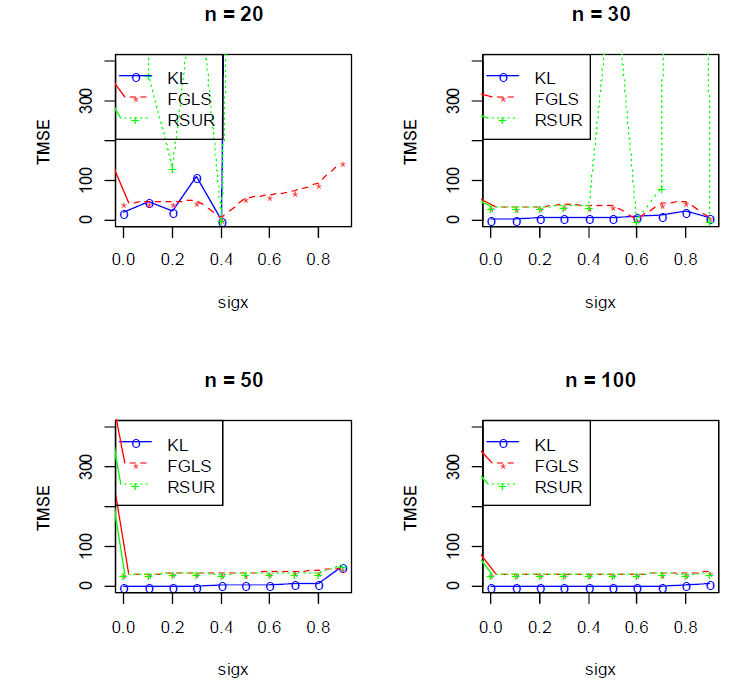# The Efficiency of the K-L Estimator for the Seemingly Unrelated Regression Model: Simulation and Application

## Authors

• Oluwayemisi Oyeronke Alaba Department of Statistics, University of Ibadan, Nigeria | Department of Mathematics and Statistics, Florida International University, Miami, FL, USA
• B. M. Golam Kibria Department of Mathematics and Statistics, Florida International University, Miami, FL, USA

## Keywords:

Multicollinearity, Ridge feasible generalized least squares estimator, Seemingly Unrelated Regression, Trace Mean Square Error, K-LSUR

## Abstract

This paper considers the Ridge Feasible Generalized Least Squares Estimator (RFGLSE), Ridge Seemingly Unrelated Regression RSUR and proposes the Kibria-Lukman KLSUR estimator for the parameters of the Seemingly Unrelated Regression (SUR) model when the regressors of the models are collinear. A simulation study was conducted to compare the performance of the three different types of estimators for the SUR model. Different correlation levels (0.0, 0.1, 0.2, ..., 0.9) among the independent variables, sample sizes replicated 10000 times and contemporaneous error correlation (0.0, 0.1, 0.2, ..., 0.9) among the equations were assumed for the simulation study. The efficiency of the three (RFGLSE, RSUR, and KLSUR estimators for SUR, when the predictors are correlated, was investigated using the Trace Mean Square Error (TMSE). The results showed that the KLSUR estimator outperformed the other estimators except for a few cases when the sample size is small.

Dimensions

A. Zellner, ”An Efficient Method of Estimating Seemingly Unrelated Regression Equations and Tests for Aggregation Bias”, Journal of the American Statistical Association 7 (1962) 48.

O. O. Alaba, O. E. Olubusoye & S. O. Ojo, ”Efficiency of Seemingly Unrelated Regression Estimator over the Ordinary Least Squares”, European Journal of Scientific Research, 39 (2010) 153.

A. Zellner & H. Theil, ”Three Stage Least Squares: Simultaneous Estimation of Simultaneous Equations”, Econometrica 30 (1962) 54.

A. Zellner, ”An Introduction to Bayesian Inference in Econometrics”, John Wiley & Sons Inc. New York, (1971).

S. B. Adebayo, ”Semi parametric Bayesian Regression for Multivariate responses”. Inaugural Dissertation zur Erlangung des Grades Doctor oeconomiae publicae (Dr. oec.publ.) an der Ludwig- Maximilians Universitat Munchen, (2003) 150.

O. O. Alaba, O. E. Olubusoye, & O. R. Oyebisi, ”Cholesky Decomposition of Variance-Covariance Matrix Effect on the Estimators of Seemingly Unrelated Regression Model”, Journal of Science Research, 12 (2013) 371.

J. Benjamin, L. Steinhardt, D. G. Walker, D. H. Peters, & D. Bishai, ”Horizontal Equity and Efficiency at Primary Health Care Facilities in Rural Afghanistan: A Seemingly Unrelated Regression Approach”, Journal of Social Science and Medicine, 89 (2013) 25.

R. Afolayan R. & B. L. Adeleke, ”On the Efficiency of Some Estimators for Modeling Seemingly Unrelated Regression with Heteroscedastic Disturbances”, Journal of Mathematics, 14 (2018) 1.

O. O. Alaba, & O. Akinrelere, ”Tests for Aggregation Bias in Seemingly Unrelated Regression with Unequal Observations”, Proceedings of the 18th ISTEAMS Multidisciplinary Cross-Border Conference (2019) 239.

O. O. Alaba & A. A. Lawal, ”Bootstrap Bartlett Adjustment on Decomposed Variance-Covariance Matrix of Seemingly Unrelated Regression Model”, Afrika Statiska 14 (2019) 1891.

O. O. Alaba, A. A. Adepoju, & O. Olaomi, ”Seemingly Unrelated Regression with Decomposed Variance-Covariance Matrix: A Bayesian Approach”, Journal of the Nigerian Association of Mathematical Physics,51 (2019) 137.

B. Yuzbasi, & S. E. Ahmed, ”Ridge Type Shrinkage Estimation of Seemingly Unrelated Regressions and Analytics of Economic and Financial Data from Fragile Five Countries”, Journal of Risk Financial Management 13 (2020) 131.

R. M. Esfanjani, D. Najarzadeh, H. J. Khamnei, F. Hormozinejad, & M. Talebi, ”Two-Parameter Ridge Estimation in Seemingly Unrelated Regression Models” Communications in Statistics - Simulation and Computation (2020).

A. F. Lukman, & K. Ayinde, ”Review and Classifications of the Ridge Parameter Estimation Technique”, Journal of Mathematics and Statistics, 46 (2017) 953.

A. E. Hoerl & R. W. Kennard, ”Ridge Regression: Biased Estimation for Nonorthogonal Problems” Technometrics 12 (1970) 55.

B. F. Swindel, ”Good Ridge Estimators based on Prior Information” Commu Stat-Theor Method, 5 (1976) 1065.

B. M. G. Kibria, ”Performance of some new ridge regression estimators”, Commun Stat-Simul Comput. 32 (2003) 419.

S. Sakallioqlu & S. Kaciranlar, ”A New Biased Estimator based on Ridge Estimation”, Stat Pap. 49 (2008) 669.

B. M. G. Kibria, & A. F. Lukman, ”A New Ridge-Type Estimator for the Linear Regression Model: Simulations and Applications”, Scientifica, (2020) 1.

O. G. Obadina, A. F. Adedotun, & O. A. Odusanya, ”Ridge Estimation’s Effectiveness for Multiple Linear Regression with Multicollinearity: An Investigation Using Monte-Carlo Simulations”, J. Nig. Soc. Phys. Sci. 3 (2021) 278.

I. Dawoud, A. F. Lukman, & A. Haadi, ”A new biased regression estimator: Theory, simulation and application”, Scientific African, 15 (2022.

G.A. Shewa, & F.I. Ugwuowo, ”A New Hybrid Estimator for Linear Regression Model Analysis: Computations and simulations”, Scientific African, 19 (2023).

W. B. Yahya, S. B. Adebayo, E. T. Jolayemi, B. A. Oyejola & O. O. M. Sanni, ”Effects of Non-Orthogonality on the Efficiency of Seemingly Unrelated Regression (SUR) Models”, Interstat Journal (2008).

A. Lawal & O. O. Alaba, ”Exploratory Analysis of Some Sectors of the Economy: A Seemingly Unrelated Regression Approach” African Journal of Applied Statistics, 6 (2019) 649.

V. K. Srivastava, & D. E. A. Giles, ”Seemingly Unrelated Regression 10

Equations” Marcel Dekker, Inc., New York (1987).

M. Alkhamisi, G. Khalaf & G. Shukur, ”Some Modifications for Choosing Ridge Parameters” Communication in Statistics Theory and Methods, 35 (2006) 2005.

M. R. Abonazel, ”A Practical Guide for Creating Monte Carlo Simulation Studies Using R”, International Journal of Mathematics and Computational Science 4 (2018) 18.

S. O. Adams, D. A. Obaromi, & A. A. Irinews, ”Goodness of Fit Test of an Autocorrelated Time Series Cubic Smoothing Spline Model” J. Nig. Soc. Phys. Sci. 3 (2021) 191.2023-06-14

## How to Cite

The Efficiency of the K-L Estimator for the Seemingly Unrelated Regression Model: Simulation and Application. (2023). Journal of the Nigerian Society of Physical Sciences, 5(3), 1414. https://doi.org/10.46481/jnsps.2023.1514

## Section

Original Research

## How to Cite

The Efficiency of the K-L Estimator for the Seemingly Unrelated Regression Model: Simulation and Application. (2023). Journal of the Nigerian Society of Physical Sciences, 5(3), 1414. https://doi.org/10.46481/jnsps.2023.1514